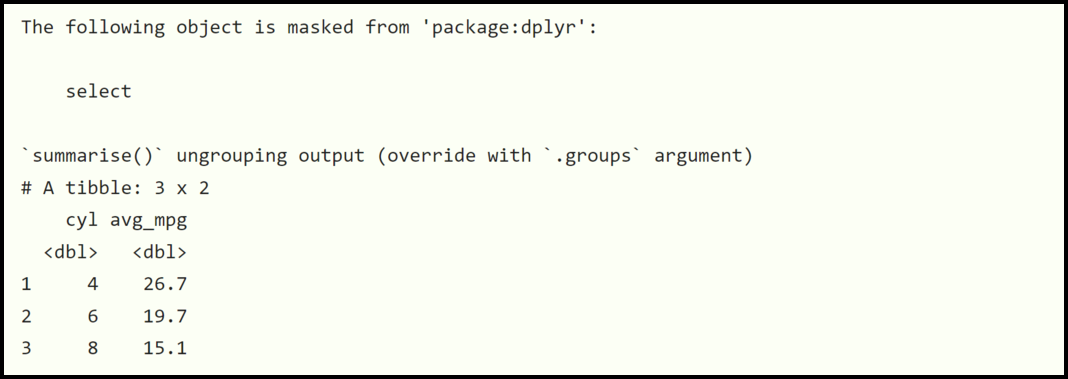GFG App
Open AppBrowser
Continue

# How to Fix: Error in select unused arguments in R?

In this article, we will be looking toward the approach to fix the error in selecting unused arguments in the R programming language.

Error in selecting unused arguments: The R compiler produces this error when a programmer tries to use the select() function of the dplyr package in R provided that the MASS package loaded. R tries to make use of the select() function from the MASS package instead when such an error takes place. This article focuses on how to fix this error.

Error: Error in select(., cyl, mpg) : unused arguments (cyl, mpg)

## When the error might occur:

Consider the following R program.

Example:

## R

 `# R program to demonstrate when the  ` `# error might occur ` ` `  `# Importing libraries ` `library``(dplyr) ` `library``(MASS) ` ` `  `# Determine the average mpg grouped by 'cyl' ` `mtcars %>% ``select``(cyl, mpg) %>% ``group_by``(cyl) %>% ``summarize``(avg_mpg = ``mean``(mpg))`

Output:Interpretation: The compiler produces this error because there is a clash between the select() function from the MASS package and the select() function from the dplyr package.

## How to Fix the Error:

This error can be eliminated by using the select() function directly from the dplyr package.

Example:

## R

 `# R program to demonstrate how to  ` `# fix the error ` ` `  `# Importing libraries ` `library``(dplyr) ` `library``(MASS) ` ` `  `# Determine the average mpg grouped by 'cyl' ` `mtcars %>% ` `  ``dplyr::``select``(cyl, mpg) %>% ` `  ``group_by``(cyl) %>% ` `  ``summarize``(avg_mpg = ``mean``(mpg)) `

Output:Interpretation: The code compiled successfully without any error because dplyr explicitly uses the select() function in the dplyr package rather than the MASS package.

My Personal Notes arrow_drop_up